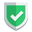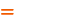我们相信：世界是美好的，你是我也是。平行空间的世界里面，不同版本的生活也在继续...

## `php`编程语言

``````function test(){
var_dump(func_num_args());
var_dump(func_get_args());
var_dump(func_get_arg(0));
}
test("a","b","c",\$d="dd","e");``````

``````int(5)
array(5) {
=>
string(1) "a"
=>
string(1) "b"
=>
string(1) "c"
=>
string(2) "dd"
=>
string(1) "e"
}
string(1) "a"``````
• 可以使用`func_get_args()`获得所有的实参。
• 可以使用`...\$args`获得剩余的所有实参。

``````function test(\$a,\$b="",...\$args){
var_dump(func_num_args());
var_dump(func_get_arg(0));
var_dump(func_get_args());
var_dump(\$a,\$args,\$b);
}
test("a","b","c",\$d="dd","e");``````

## `python`编程语言

``````def test(aa,*args,**kwargs):
print(args,args)          # ('b', 'c') b
print(kwargs,kwargs["a2"])   # {'a2': 'a2'} a2
test("a","b","c",a2="a2")
print(test.__code__.co_argcount) # 1
print(test.__code__.co_varnames) # ('aa', 'args', 'kwargs')``````

``````('b', 'c') b
{'a2': 'a2'} a2
1
('aa', 'args', 'kwargs')
None``````

`python`代码里面，目前使用`*args``**kwargs`可以获得所有剩余实参，通过`.__code__.co_argcount``.__code__.co_varnames`，获得是形参的相关情况，而不是实参。

## `node`编程语言

``````function test(a,...args){
console.log(args,args)
}
test("a","b","c",d="dd","e");``````

``[ 'b', 'c', 'dd', 'e' ] b``

``````const test = function (...args) {
console.log(...args, args, args);
}
test("sunan", "苏南", { "苏南大叔": "wonderful" });``````

``sunan 苏南 { '苏南大叔': 'wonderful' } sunan 苏南``

``````function test() {
console.log(arguments,arguments);
}
test(1, 2, 3);``````

``````[Arguments] { '0': 1, '1': 2, '2': 3 }
1``````

``````const test = () => {
console.log(arguments);
}
test("苏南","大叔");

function test2() {
console.log(arguments,arguments);
}
test2("苏南","大叔");``````

## 表格对比

php`func_get_args()``...\$args`形参`\$d=""`中的`\$d`暂时没用
python`*args,**kwargs`形参`\$d=""``**kwargs`捕获
node/js`...args`形参`d=""`中的`d`暂时没用
node/js`arguments`个别情况下，`arguments`变量符合要求

## 总结如果本文对您有帮助，或者节约了您的时间，欢迎打赏瓶饮料，建立下友谊关系。本博客不欢迎：各种镜像采集行为。请尊重原创文章内容，转载请保留作者链接。【福利】 腾讯云最新爆款活动！1核2G云服务器首年50元！【源码】本文代码片段及相关软件，请点此获取更多信息【绝密】秘籍文章入口，仅传授于有缘之人php    python